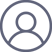姬扬2019-11-7 15:40

$U(P)=\int_{\Sigma} \frac{A \mathrm{e}^{i kr^{\prime}}}{r^{\prime}} \frac{\mathrm{e}^{ik r}}{r} \frac{-\mathrm{i}}{\lambda} \cdot \frac{1}{2}\left[\cos \left(\hat{n}, r^{\prime}\right)-\cos (\hat{n}, r)\right] \mathrm{d} \Sigma$$r=d+x^2/2d+x^2/2R=d(1+ \frac{R+d}{2R}x^2)$选取$R,d\gg \lambda$，就有$r\gg\lambda$，尽管$r$的变化并不大，但$\mathrm{e}^{i kr}$是高速振荡的，也就是说，只有在$Q$点附近的球面波前才对$P$的振幅有贡献。因此，倾斜因子就可以近似为1，而分母里的$r$也可以用$d$来代替，

$U(P)\sim \int_{\Sigma} \frac{\mathrm{e}^{ik r}}{r} \frac{-\mathrm{i}}{\lambda} \mathrm{d} \Sigma \sim \int \frac{\mathrm{e}^{ikd(1+x^2 \frac{R+d}{2R})}}{d} \frac{-\mathrm{i}}{\lambda} 2\pi x\mathrm{d}x$

$\int \mathrm{e}^{i x^2/2} x\mathrm{d}x = \frac{\mathrm{e}^{i x^2/2}}{i}$